# Canvas Basics – Arkanoid Game TutorialWhether you know it as Breakout, Arkanoid or any of the many names the game is known as, this tutorial will show you how to create Arkanoid using just a simple text editor and web browser.

Over the last few posts, we have been looking at elements of code for gaming and we even created a tennis-style game like Pong.

We will now create a game we are going to call Brickbreaker for no other reason than we have to call it something.

In this post I will piece together the game, a lot of it will come from code covered in earlier posts, some of it may be new and will be explained.

You can find a finished version of this game here http://games.thejaytray.com/brickbreaker.html, in later posts we will cover other elements like ending the game, going to a new level, etc..  The version of the game we are looking at here is to apply what has been covered so far.

## Assumptions

I am working off the assumption that you have either a basic (at least) understanding of HTML 5 and Canvas/JavaScript, or that you have been following these posts:

The code you are about to see will include elements of the previous posts, plus some extra bits that will be explained as we go along.

## HTML File

In your text editor, create a new file called “brickbreaker.html”.

You will create this file in your text editor and then close it, you will view this file using a text editor then.

If following the posts so far, you will already have a page with this code: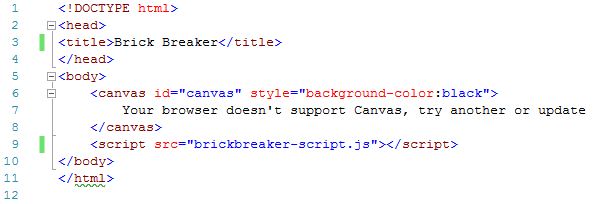```<!DOCTYPE html>
<title>Brick Breaker</title>
<body>
<canvas id="canvas" style="background-color:black">
Your browser doesn't support Canvas, try another or update
</canvas>
<script src="brickbreaker-script.js"></script>
</body>
</html>
```

## JavaScript File

In your text editor, create a file called “brickbreaker-script.js” and save it to the same location as your html file.

We will create a 2d canvas with a width of 670 and height of 400.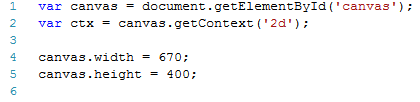```var canvas = document.getElementById('canvas');
var ctx = canvas.getContext('2d');
canvas.width = 670;
canvas.height = 400;
```

## Breakout & Arkanoid Game Tutorial

Now that we have the basic canvas area set up, let’s start building the game.

We will add variables for each element of the game, then the functions to draw, move and detect collisions.

The end result will be the basic game, there will be some parts still missing that we may cover in later posts, these elements include detecting when all bricks have been cleared, power ups, etc.

## Brick Variables

The values for our bricks will include the number of rows and columns of bricks; the height and width of bricks; the padding and spacing around the bricks; and we are also going to randomly select RGB values for the fill color of the bricks.```var bRowCount = 5;
var bColCount = 10;
var bHeight = 20;
var bWidth = 60;
var bTopOffset = 10;
var bLeftOffset = 10;
var myRed = Math.floor(Math.random() * 256);
var myGreen = Math.floor(Math.random() * 256);
var myBlue = Math.floor(Math.random() * 256);
```

In your version of the game, you don’t have to randomly select RGB values, when drawing the bricks you can enter a color value then if you wanted.

After going through this code, you might choose to do that to see the effects yourself.

## Ball Variables

Unlike the ball in our last Pong game, in this game the ball will be…. well…. ball-shaped.

This time we will have a Radius value (ballR), we also have starting X and Y positions.

The speed the ball moves vertically (up/down) will be called vertiSpeed and the horizontal (left/right) speed will be called horiSpeed.

The pBallAngle will be a calculation we will come across later.  If the ball had the same horiSpeed every time, you will essentially be playing the same game over and over as the ball follows the same path every time and the angle doesn’t change.

What we are going to do later is change the horiSpeed based on how far from the center of the paddle the ball hits the paddle.  This will allow for changing horiSpeed, which looks like the angle is changing.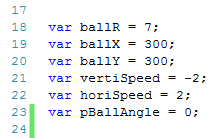```var ballR = 7;
var ballX = 300;
var ballY = 300;
var vertiSpeed = -2;
var horiSpeed = 2;
var pBallAngle = 0;
```

The Paddle variables should be fairly familiar to you at this point; there are X and Y positions; width and height values; fill color and a speed.

Note the speed the paddle moves at should be at least as fast as the ball will move, otherwise you could be playing a very short and annoying game!```var pX = 300;
var pY = 380;
var pWidth = 60;
var pHeight = 10;
var pColor = '#FFFFFF';
var pSpeed = 5;
```

## Other Variables & Key EventListener

The last two variables to be set up are both arrays, one for storing the keys pressed and released and also to store the bricks we will be creating.

Then we have the two event listeners for when keys are pressed and released.```var keys = {};
var bricks = {};
for (c = 0; c < bColCount; c++) {
bricks[c] = {};
for (r = 0; r < bRowCount; r++) {
bricks[c][r] = { x: 0, y: 0, status: 1 };
}
}
keys[e.keyCode] = true;
e.preventDefault();
});
delete keys[e.keyCode];
});
```

## Draw Ball Function

Since we have yet to create a ball or circle in these posts, I will spend a bit more time on this function than I will be on other functions already covered.The drawBall function will draw a circle, this circle will be the ball in our game.

1. ctx.beginPath(); We are going to start a path for a type of line
2. ctx.arc(ballX, ballY, ballR, 0, 2 * Math.PI); Our path is going to be an arc (or curved line).  The X and Y variables related to the middle of our circle.  ballR is a variable we set earlier and will be the radius of our circle.  The next parameter is the starting angle (in radians) of our path, we are starting at zero in our circle, this is the 3o’clock position if you imagine a clock face.  The last parameter is the ending position, in radians, of our arc.  A full circle is 2 * PI.
3. ctx.fillStyle = “#AA55DD”; The fill color for our ball.
4. ctx.fill(); Fill in our circle
5. ctx.closePath(); The closePath method creates a path from the current point to the starting point of our path.  In our case we have created a full circle and close the path now.

The full drawBall functions is:

```function drawBall() {
ctx.beginPath();
ctx.arc(ballX, ballY, ballR, 0, 2 * Math.PI);
ctx.fillStyle = "#AA55DD";
ctx.fill();
ctx.closePath();
}
```

Next we will draw our paddle which will be controlled by the player.

In earlier posts we had a box function that we used to base other box objects on, this time we are going to draw a rectangle using the beginPath and closePath methods.

For the ball we created an arc, now we are gong to create a rect (rectangle).

This uses the variables set earlier in this post.```function drawPaddle() {
ctx.beginPath();
ctx.rect(pX, pY, pWidth, pHeight);
ctx.fillStyle = pColor;
ctx.fill();
ctx.closePath();
}
```

## Draw Bricks Function

The drawBricks function is similar to the one created in the last post.

There is one difference, the fillStyle is using the random numbers from the variables set up earlier.  In the last post, these random numbers were selected within the function.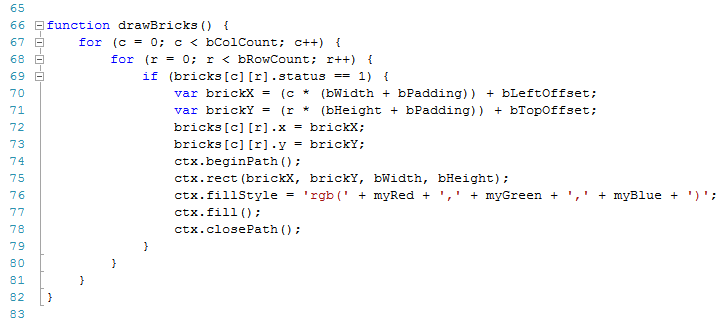This function creates an array of bricks.

It goes through the assigned number of columns and rows of bricks and sets the status of each brick to be 1 (which will mean the brick gets drawn).

The  X and Y values of each brick is calculated based on the padding, offset and dimensions of the bricks.

The code of the drawBricks functions is:

```function drawBricks() {
for (c = 0; c < bColCount; c++) {
for (r = 0; r < bRowCount; r++) {
if (bricks[c][r].status == 1) {
var brickX = (c * (bWidth + bPadding)) + bLeftOffset;
var brickY = (r * (bHeight + bPadding)) + bTopOffset;
bricks[c][r].x = brickX;
bricks[c][r].y = brickY;
ctx.beginPath();
ctx.rect(brickX, brickY, bWidth, bHeight);
ctx.fillStyle = 'rgb(' + myRed + ',' + myGreen + ',' + myBlue + ')';
ctx.fill();
ctx.closePath();
}
}
}
}
```

## Collision Detection Function

Lines 85 to 88 of our code will work through each individual brick in our array that has a status of 1.

Then line 89 is going to check if the ball is in the same position as any of these bricks.How does line 89 work? Let’s break it down and use a basic example to demonstrate.

In the image below, the blue area is greater than the dotted line which we will call “x1”.In the image below, the blue area is greater than x1 and also less then the next dotted line which we will call x2 in this example.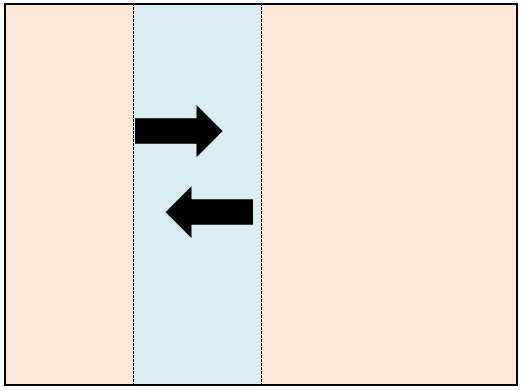In the image below, there is a 3rd dotted line, Y1.

The area in blue is greater than x1 AND less than y2 and greater than y1.And finally we add a 4th dotted line, y2.

The blue area below is greater than x1 AND less than x2 AND greater than y1 AND less than y2.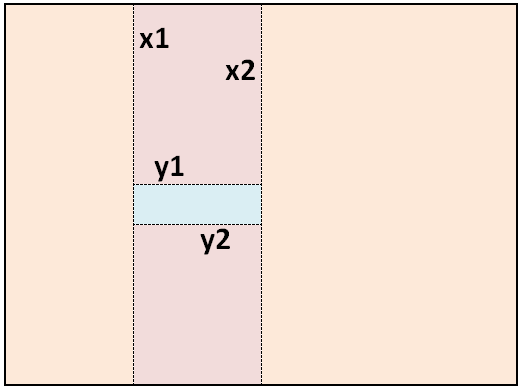If you think of the blue area above as being our paddle, what our collision detection is doing is checking if the ball is within this “blue” area for each of the bricks.

The collisiondetection function is:

```function collisionDetection() {
for (c = 0; c < bColCount; c++) {
for (r = 0; r < bRowCount; r++) {
var b = bricks[c][r];
if (b.status == 1) {
if (ballX > b.x && ballX < b.x + bWidth && ballY > b.y && ballY < b.y + bHeight) {
vertiSpeed = -vertiSpeed;
b.status = 0;
}
}
}
}
}
```

## Draw Function

This draw function will be our longest function so we will break it down into smaller pieces.We start by clearing the canvas area every time the code updates

We then call the drawBricks, drawPaddle, drawBall and collisionDetection functions.Lines 104 to 109 give instructions on what to do when the ball hits the left or right sides and when the ball hits the top.

Lines 104 and 105 changes the horizontal (left/right) direction of the ball if it hits the right side OR the left side of the canvas.

Lines 107 and 108 change the vertical (up/down) direction of the ball if it hits the top of the canvas area.Lines 110 to 112 check if the ball has collided with the paddle.

Then line 113 calculates a value for our pBallAngle variable.

This looks at the difference between the ball’s X value and the X value of the middle of the paddle.

This difference is then multiplied by 0.05 and in line 114, this is added to the horiSpeed to effectively change the angle the ball moves at, based on how far to the left or right hand side of the paddle the ball collides.

Change the 0.05 value when you finish the game to see the difference this makes.

I deliberately made it a small value because I don’t want the ball moving faster than my paddle can.

Lines 114 and 115 change the direction the ball is moving at.

In lines 118 we are saying if none of the conditions of the ball already set is true, that is basically to say, if the ball has gone below paddle level and not hit the paddle, then in line 119 we want an alert message to say “GAME OVER”.

When playing the game, this alert will include an OK button, when you click it the page reloads.Lines 123 to 131 handles the key events for the left and right arrow keys.

Lines 132 and 133 then update the ball’s X and Y  values.

The full draw function is:

```function draw() {
ctx.clearRect(0, 0, canvas.width, canvas.height);
drawBricks();
drawBall();
collisionDetection();
if(ballX + horiSpeed > canvas.width-ballR || ballX + horiSpeed < ballR) {
horiSpeed = -horiSpeed;
}
if(ballY + vertiSpeed < ballR) {
vertiSpeed = -vertiSpeed;
}
else if (ballY + vertiSpeed> pY - ballR ) {
if (ballX + ballR > pX && ballX -ballR < pX + pWidth) {
if (ballY = ballY - pHeight) {
pBallAngle = ((pX + pWidth / 2) - ballX) * 0.05;
horiSpeed = horiSpeed + pBallAngle;
vertiSpeed = -vertiSpeed;
}
}
else {
}
}
if (37 in keys) {
if (pX - pSpeed > 0) {
pX -= pSpeed;
}
} else if (39 in keys) {
if (pX + pWidth + pSpeed < canvas.width) {
pX += pSpeed;
}
}
ballX += horiSpeed;
ballY += vertiSpeed;
}

```

## Loop Function

Our last function is the loop function which calls the draw function and includes the requestAnimationFrame.The last line of the script calls the loop function.

## Breakout – Arkanoid Game Tutorial Full Script

The full script for this game is as follows:

```var canvas = document.getElementById('canvas');
var ctx = canvas.getContext('2d');
canvas.width = 670;
canvas.height = 400;
var bRowCount = 5;
var bColCount = 10;
var bHeight = 20;
var bWidth = 60;
var bTopOffset = 10;
var bLeftOffset = 10;
var myRed = Math.floor(Math.random() * 256);
var myGreen = Math.floor(Math.random() * 256);
var myBlue = Math.floor(Math.random() * 256);
var ballR = 7;
var ballX = 300;
var ballY = 300;
var vertiSpeed = -2;
var horiSpeed = 2;
var pBallAngle = 0;
var pX = 300;
var pY = 380;
var pWidth = 60;
var pHeight = 10;
var pColor = '#FFFFFF';
var pSpeed = 5;
var keys = {};
var bricks = {};
for (c = 0; c < bColCount; c++) {
bricks[c] = {};
for (r = 0; r < bRowCount; r++) {
bricks[c][r] = { x: 0, y: 0, status: 1 };
}
}
keys[e.keyCode] = true;
e.preventDefault();
});
delete keys[e.keyCode];
});
function drawBall() {
ctx.beginPath();
ctx.arc(ballX, ballY, ballR, 0, 2 * Math.PI);
ctx.fillStyle = "#AA55DD";
ctx.fill();
ctx.closePath();
}
ctx.beginPath();
ctx.rect(pX, pY, pWidth, pHeight);
ctx.fillStyle = pColor;
ctx.fill();
ctx.closePath();
}
function drawBricks() {
for (c = 0; c < bColCount; c++) {
for (r = 0; r < bRowCount; r++) {
if (bricks[c][r].status == 1) {
var brickX = (c * (bWidth + bPadding)) + bLeftOffset;
var brickY = (r * (bHeight + bPadding)) + bTopOffset;
bricks[c][r].x = brickX;
bricks[c][r].y = brickY;
ctx.beginPath();
ctx.rect(brickX, brickY, bWidth, bHeight);
ctx.fillStyle = 'rgb(' + myRed + ',' + myGreen + ',' + myBlue + ')';
ctx.fill();
ctx.closePath();
}
}
}
}
function collisionDetection() {
for (c = 0; c < bColCount; c++) {
for (r = 0; r < bRowCount; r++) {
var b = bricks[c][r];
if (b.status == 1) {
if (ballX > b.x && ballX < b.x + bWidth && ballY > b.y && ballY < b.y + bHeight) {
vertiSpeed = -vertiSpeed;
b.status = 0;
}
}
}
}
}
function draw() {
ctx.clearRect(0, 0, canvas.width, canvas.height);
drawBricks();
drawBall();
collisionDetection();
if(ballX + horiSpeed > canvas.width-ballR || ballX + horiSpeed < ballR) {
horiSpeed = -horiSpeed;
}
if(ballY + vertiSpeed < ballR) {
vertiSpeed = -vertiSpeed;
}
else if (ballY + vertiSpeed> pY - ballR ) {
if (ballX + ballR > pX && ballX -ballR < pX + pWidth) {
if (ballY = ballY - pHeight) {
pBallAngle = ((pX + pWidth / 2) - ballX) * 0.05;
horiSpeed = horiSpeed + pBallAngle;
vertiSpeed = -vertiSpeed;
}
}
else {
}
}
if (37 in keys) {
if (pX - pSpeed > 0) {
pX -= pSpeed;
}
} else if (39 in keys) {
if (pX + pWidth + pSpeed < canvas.width) {
pX += pSpeed;
}
}
ballX += horiSpeed;
ballY += vertiSpeed;
}
function loop() {
draw();
window.requestAnimationFrame(loop);
}
loop();
```

## Try It Yourself

Change some of the variables, colors, speeds and pBallAngle calculations, save the changes in your text editor and refresh your browser.

Could you add in code to end the game when all bricks have been cleared?

Can you improve the collision detection and angle the ball bounces back at when it hits the paddle?

Can you set up some of the bricks to need to be hit twice before disappearing?

There are many changes and improvements that can be made to the game as it is now and we will cover some of these improvements as we continue going through these posts.

## Disclaimer

I am not claiming that the code that I use in these examples is the best, most efficient and up to date code.

Code gets updated and improved over time, what is best practice today may not be tomorrow.

Follow